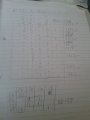# Hey Guys. Im working with Karnaugh Mapping and Digital Logic and need some help.

#### DanRab

Joined May 4, 2015
8
The problem was : Z(A, B, C, D) = ∑m(0,2,5,7,8,10,13,15) do the following: i) Represent the logic equation in a Karnaugh map.  ii) Determine the most simplified equation and implement the resulting circuit using basic logic gates. 

I managed to simplify the logic into a karnaugh map and was left with the equation BD+B'D'.
Im not sure on how to implement this with basic logic gates.

Thank you.#### tshuck

Joined Oct 18, 2012
3,534
You've gotten the harder part. Try writing out, in words, what the resulting expression means. This describes the logical interconnections for implementing the expression using gates.

If you need more reading material, you can find some in the eBook.

As an aside, BD+B'D' is not an equation - it needs an equals sign for that. Z = BD+B'D' is an equation.

#### DanRab

Joined May 4, 2015
8

#### WBahn

Joined Mar 31, 2012
26,398
There's also the question of what it means to be "most simplified", something that few texts or instructors bother to recognize. This equation can be implemented using a single two-input standard logic gate, but that is probably not what is being asked for.

#### shteii01

Joined Feb 19, 2010
4,644
That does not sound right.
You don't simplify logic into Karnaugh Map.
You put the logic into the map, then use the map to simplify the logic. The map shows which parts of the logic you, the user, don't need.

#### WBahn

Joined Mar 31, 2012
26,398
That does not sound right.
You don't simplify logic into Karnaugh Map.
You put the logic into the map, then use the map to simplify the logic. The map shows which parts of the logic you, the user, don't need.
Looking at his work, I think he did it the usual way. His word choice in the verbal description just made it seem backwards.

But I sure do applaud him for showing his work in the original post!